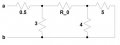# Circuit Analysis - find resistance value

#### juanjuan

Joined Oct 24, 2013
17
Hello everyone. I need some help with this exercise. I need to know what is the value of R_0 when the resistance between a and b is 2 ohm

I can't upload a picture of the circuit, so I uploaded it here:I couldn't go to this class, that's why I don't know how to solve it. They used a method (in class they call it "Pro Method") that consists in "deciding" the value of one current and solve the exercise using that value as a known value, do you understand? Do you know what is the name of that method (it's not "pro method")?

Thanks

Moderators note : uploaded and inserted pucture

Last edited by a moderator:

#### WBahn

Joined Mar 31, 2012
25,757
I don't know what method they might be talking about. I can't think of any that sound like "pro method".

Q1) What is the total current flowing into the circuit from Node B?

Q2) What is the voltage at the left side of R_0?

Q3) What is the current flowing downward in the 3Ω resistor?

Q4) What is the current flowing left-to-right through R_0?

Q5) What is the current flowing out of R_0 into the parallel combination of the 4Ω and 5Ω resistors?

Q6) What is the effective resistance of the parallel combination of the 4Ω and 5Ω resistors?

Q7) What is the voltage at the right side of R_0?

Q8) What is the voltage across R_0?

Q9) In light of the answers to Q4 and Q8, what is the value of R_0?

#### WBahn

Joined Mar 31, 2012
25,757
Another way to approach it is to partition the circuit and see what has to be true for the portion that still contains R_0.

For instance, if we partition the circuit into the 0.5Ω resistor and everything else, what does the resistance of "everything else" need to be in order for the total resistance of the combined circuit to be 2Ω. Once you have that number, then you can partition the circuit into the part that has the 3Ω resistor and "everything else" and ask what the resistance of the new "everything else" needs to be in order for the combined circuit (these two partitions) to be whatever the result was from the first step. Then you proceed to partition the new "everything else" again.

#### juanjuan

Joined Oct 24, 2013
17

#### juanjuan

Joined Oct 24, 2013
17
I solved it, but I think not in the most effective way. I used parallel and series resistance and I got the correct answer

#### WBahn

Joined Mar 31, 2012
25,757
Glad you solved it. Unless you show us your work, we can't possibly comment on the effectiveness of your approach.